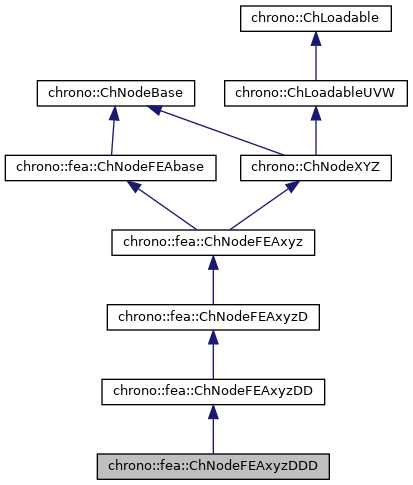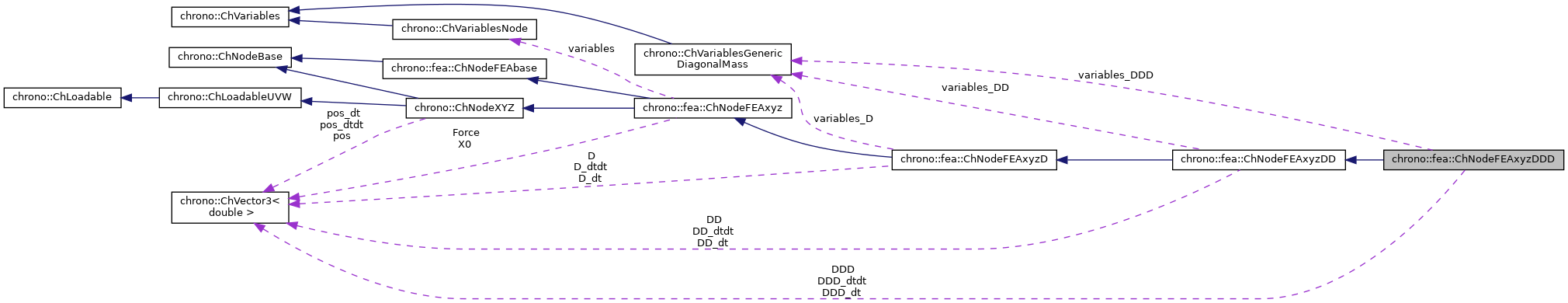chrono::fea::ChNodeFEAxyzDDD Class Reference

## Description

Class for a generic 3D finite element node, with x,y,z displacement, and 3 position vector derivatives.

For a Fully Parameterized ANCF element:

• The variable D represents the position vector gradient with respect to the 1st element coordinate line.
• The variable DD represents the position vector gradient with respect to the 2nd element coordinate line.
• The variable DDD represents the position vector gradient with respect to the 3rd element coordinate line. Other ANCF elements may use these derivative vectors differently.

#include <ChNodeFEAxyzDDD.h>

Inheritance diagram for chrono::fea::ChNodeFEAxyzDDD:[legend]
Collaboration diagram for chrono::fea::ChNodeFEAxyzDDD:[legend]

## Public Member Functions

ChNodeFEAxyzDDD (ChVector<> initial_pos=VNULL, ChVector<> initial_dir_u=VECT_X, ChVector<> initial_dir_v=VECT_Y, ChVector<> initial_dir_w=VECT_Z)

ChNodeFEAxyzDDD (const ChNodeFEAxyzDDD &other)

ChNodeFEAxyzDDDoperator= (const ChNodeFEAxyzDDD &other)

void SetDDD (const ChVector<> &d)
Set the 3rd derivative vector.

const ChVectorGetDDD () const
Get the 3rd derivative vector.

void SetDDD_dt (const ChVector<> &dt)
Set the speed of the 3rd derivative vector.

const ChVectorGetDDD_dt () const
Get the speed of the 3rd derivative vector.

void SetDDD_dtdt (const ChVector<> &dtt)
Set the acceleration of the 3rd derivative vector.

const ChVectorGetDDD_dtdt () const
Get the acceleration of the 3rd derivative vector.

ChVariablesVariables_DDD ()

virtual void SetNoSpeedNoAcceleration () override
Reset to no speed and acceleration.

ChVectorDynamicGetMassDiagonalDDD ()
Get mass of the node (for DDD variables).

virtual void SetFixed (bool fixed) override
Fix/release this node. More...

virtual bool IsFixed () const override
Return true if the node is fixed (i.e., its state variables are not changed by the solver).

void SetFixedDDD (bool fixed)
Fix/release the 3rd derivative vector states. More...

bool IsFixedDDD () const
Return true if the 3rd derivative vector states are fixed.

virtual int GetNdofX () const override
Get the number of degrees of freedom.

virtual int GetNdofW () const override
Get the number of degrees of freedom, derivative.

virtual int GetNdofX_active () const override
Get the actual number of active degrees of freedom.

virtual int GetNdofW_active () const override
Get the actual number of active degrees of freedom, derivative.

virtual void NodeIntStateGather (const unsigned int off_x, ChState &x, const unsigned int off_v, ChStateDelta &v, double &T) override

virtual void NodeIntStateScatter (const unsigned int off_x, const ChState &x, const unsigned int off_v, const ChStateDelta &v, const double T) override

virtual void NodeIntStateGatherAcceleration (const unsigned int off_a, ChStateDelta &a) override

virtual void NodeIntStateScatterAcceleration (const unsigned int off_a, const ChStateDelta &a) override

virtual void NodeIntStateIncrement (const unsigned int off_x, ChState &x_new, const ChState &x, const unsigned int off_v, const ChStateDelta &Dv) override

virtual void NodeIntStateGetIncrement (const unsigned int off_x, const ChState &x_new, const ChState &x, const unsigned int off_v, ChStateDelta &Dv) override

virtual void NodeIntLoadResidual_F (const unsigned int off, ChVectorDynamic<> &R, const double c) override

virtual void NodeIntLoadResidual_Mv (const unsigned int off, ChVectorDynamic<> &R, const ChVectorDynamic<> &w, const double c) override

virtual void NodeIntToDescriptor (const unsigned int off_v, const ChStateDelta &v, const ChVectorDynamic<> &R) override

virtual void NodeIntFromDescriptor (const unsigned int off_v, ChStateDelta &v) override

virtual void InjectVariables (ChSystemDescriptor &descriptor) override
Tell to a system descriptor that there are variables of type ChVariables in this object (for further passing it to a solver).

virtual void VariablesFbReset () override
Set the 'fb' part (the known term) of the encapsulated ChVariables to zero.

virtual void VariablesFbLoadForces (double factor=1) override
Add the current forces (applied to node) into the encapsulated ChVariables. More...

Initialize the 'qb' part of the ChVariables with the current value of speeds.

virtual void VariablesQbSetSpeed (double step=0) override
Fetch the item speed (ex. More...

virtual void VariablesFbIncrementMq () override
Add M*q (masses multiplied current 'qb') to Fb, ex. More...

virtual void VariablesQbIncrementPosition (double step) override
Increment node positions by the 'qb' part of the ChVariables, multiplied by a 'step' factor. More...

Gets the number of DOFs affected by this element (position part).

Gets the number of DOFs affected by this element (speed part).

virtual void LoadableGetStateBlock_x (int block_offset, ChState &S) override
Gets all the DOFs packed in a single vector (position part).

virtual void LoadableGetStateBlock_w (int block_offset, ChStateDelta &S) override
Gets all the DOFs packed in a single vector (speed part).

virtual void LoadableStateIncrement (const unsigned int off_x, ChState &x_new, const ChState &x, const unsigned int off_v, const ChStateDelta &Dv) override
Increment all DOFs using a delta.

virtual int Get_field_ncoords () override
Number of coordinates in the interpolated field.

virtual unsigned int GetSubBlockSize (int nblock) override
Get the size of the i-th sub-block of DOFs in global vector.

virtual void LoadableGetVariables (std::vector< ChVariables * > &vars) override
Get the pointers to the contained ChVariables, appending to the mvars vector.

virtual void ComputeNF (const double U, const double V, const double W, ChVectorDynamic<> &Qi, double &detJ, const ChVectorDynamic<> &F, ChVectorDynamic<> *state_x, ChVectorDynamic<> *state_w) override
Evaluate Q = N'*F, for Q generalized lagrangian load, where N is some type of matrix evaluated at point P(U,V,W) assumed in absolute coordinates, and F is a load assumed in absolute coordinates. More...

virtual void ArchiveOUT (ChArchiveOut &archive) override
Method to allow serialization of transient data to archives.

virtual void ArchiveIN (ChArchiveIn &archive) override
Method to allow de serialization of transient data from archives.Public Member Functions inherited from chrono::fea::ChNodeFEAxyzDD
ChNodeFEAxyzDD (ChVector<> initial_pos=VNULL, ChVector<> initial_dir=VECT_X, ChVector<> initial_curv=VNULL)

ChNodeFEAxyzDD (const ChNodeFEAxyzDD &other)

ChNodeFEAxyzDDoperator= (const ChNodeFEAxyzDD &other)

void SetDD (const ChVector<> &d)
Set the 2nd derivative vector.

const ChVectorGetDD () const
Get the 2nd derivative vector.

void SetDD_dt (const ChVector<> &dt)
Set the speed of the 2nd derivative vector.

const ChVectorGetDD_dt () const
Get the speed of the 2nd derivative vector.

void SetDD_dtdt (const ChVector<> &dtt)
Set the acceleration of the 2nd derivative vector.

const ChVectorGetDD_dtdt () const
Get the acceleration of the 2nd derivative vector.

ChVariablesVariables_DD ()

ChVectorDynamicGetMassDiagonalDD ()
Get mass of the node (for DD variables).

void SetFixedDD (bool fixed)
Fix/release the 2nd derivative vector states. More...

bool IsFixedDD () const
Return true if the 2nd derivative vector states are fixed.Public Member Functions inherited from chrono::fea::ChNodeFEAxyzD
ChNodeFEAxyzD (ChVector<> initial_pos=VNULL, ChVector<> initial_dir=VECT_X)

ChNodeFEAxyzD (const ChNodeFEAxyzD &other)

ChNodeFEAxyzDoperator= (const ChNodeFEAxyzD &other)

void SetD (const ChVector<> &d)
Set the derivative vector.

const ChVectorGetD () const
Get the derivative vector.

void SetD_dt (const ChVector<> &dt)
Set the speed of the derivative vector.

const ChVectorGetD_dt () const
Get the speed of the derivative vector.

void SetD_dtdt (const ChVector<> &dtt)
Set the acceleration of the derivative vector.

const ChVectorGetD_dtdt () const
Get the acceleration of the derivative vector.

ChVariablesVariables_D ()

ChVectorDynamicGetMassDiagonalD ()
Get mass of the node.

void SetFixedD (bool fixed)
Fix/release the derivative vector states. More...

bool IsFixedD () const
Return true if the derivative vector states are fixed.Public Member Functions inherited from chrono::fea::ChNodeFEAxyz
ChNodeFEAxyz (ChVector<> initial_pos=VNULL)

ChNodeFEAxyz (const ChNodeFEAxyz &other)

ChNodeFEAxyzoperator= (const ChNodeFEAxyz &other)

virtual ChVariablesNodeVariables () override

virtual void Relax () override
Set the rest position as the actual position.

virtual double GetMass () const override
Get mass of the node.

virtual void SetMass (double m) override
Set mass of the node.

virtual void SetX0 (ChVector<> x)
Set the initial (reference) position.

virtual ChVector GetX0 ()
Get the initial (reference) position.

virtual void SetForce (ChVector<> f)
Set the 3d applied force, in absolute reference.

virtual ChVector GetForce ()
Get the 3d applied force, in absolute reference.

virtual ChVariablesGetVariables1 () overridePublic Member Functions inherited from chrono::fea::ChNodeFEAbase
virtual void SetIndex (unsigned int mindex)
Sets the global index of the node.

virtual unsigned int GetIndex ()
Gets the global index of the node.Public Member Functions inherited from chrono::ChNodeBase
ChNodeBase (const ChNodeBase &other)

ChNodeBaseoperator= (const ChNodeBase &other)

virtual bool UseFullDof () const
Return true if all node DOFs are active (no node variable is fixed).

unsigned int NodeGetOffsetX ()
Get offset in the state vector (position part).

unsigned int NodeGetOffsetW ()
Get offset in the state vector (speed part).

void NodeSetOffset_x (const unsigned int moff)
Set offset in the state vector (position part).

void NodeSetOffset_w (const unsigned int moff)
Set offset in the state vector (speed part).Public Member Functions inherited from chrono::ChNodeXYZ
ChNodeXYZ (const ChVector<> &initial_pos)

ChNodeXYZ (const ChNodeXYZ &other)

ChNodeXYZoperator= (const ChNodeXYZ &other)

const ChVectorGetPos () const

void SetPos (const ChVector<> &mpos)

const ChVectorGetPos_dt () const

void SetPos_dt (const ChVector<> &mposdt)

const ChVectorGetPos_dtdt () const

void SetPos_dtdt (const ChVector<> &mposdtdt)

virtual int GetSubBlocks () override
Get the number of DOFs sub-blocks.

virtual unsigned int GetSubBlockOffset (int nblock) override
Get the offset of the specified sub-block of DOFs in global vector.

virtual bool IsSubBlockActive (int nblock) const override
Check if the specified sub-block of DOFs is active.

virtual double GetDensity () override
This is not needed because not used in quadrature.Public Member Functions inherited from chrono::ChLoadableUVW
virtual bool IsTetrahedronIntegrationNeeded ()
If true, use quadrature over u,v,w in [0..1] range as tetrahedron volumetric coords (with z=1-u-v-w) otherwise use default quadrature over u,v,w in [-1..+1] as box isoparametric coords.

virtual bool IsTrianglePrismIntegrationNeeded ()
If true, use quadrature over u,v in [0..1] range as triangle natural coords (with z=1-u-v), and use linear quadrature over w in [-1..+1], otherwise use default quadrature over u,v,w in [-1..+1] as box isoparametric coords.

## Protected Member Functions

virtual void SetupInitial (ChSystem *system) override
Initial setup. Set number of degrees of freedom for this node.Public Types inherited from chrono::ChVariableTupleCarrier_1vars< 3 >
typedef ChConstraintTuple_1vars< ChVariableTupleCarrier_1vars< N1 > > type_constraint_tuplePublic Attributes inherited from chrono::fea::ChNodeFEAbase
double m_TotalMass
Nodal mass obtained from element masss matrix.Public Attributes inherited from chrono::ChNodeXYZ
ChVector pos

ChVector pos_dt

ChVector pos_dtdtStatic Public Attributes inherited from chrono::ChVariableTupleCarrier_1vars< 3 >
static const int nvars1Protected Attributes inherited from chrono::fea::ChNodeFEAxyzD
int m_dof_actual
actual number of degrees of freedomProtected Attributes inherited from chrono::fea::ChNodeFEAxyz
ChVariablesNode variables
3D node variables, with x,y,z

ChVector X0
reference position

ChVector Force
applied forceProtected Attributes inherited from chrono::fea::ChNodeFEAbase
unsigned int g_index
global node indexProtected Attributes inherited from chrono::ChNodeBase
unsigned int offset_x
offset in vector of state (position part)

unsigned int offset_w
offset in vector of state (speed part)

## ◆ ComputeNF()

 void chrono::fea::ChNodeFEAxyzDDD::ComputeNF ( const double U, const double V, const double W, ChVectorDynamic<> & Qi, double & detJ, const ChVectorDynamic<> & F, ChVectorDynamic<> * state_x, ChVectorDynamic<> * state_w )
overridevirtual

Evaluate Q = N'*F, for Q generalized lagrangian load, where N is some type of matrix evaluated at point P(U,V,W) assumed in absolute coordinates, and F is a load assumed in absolute coordinates.

Here, det[J] is unused.

Parameters
 U x coordinate of application point in absolute space V y coordinate of application point in absolute space W z coordinate of application point in absolute space Qi Return result of N'*F here, maybe with offset block_offset detJ Return det[J] here F Input F vector, containing Force xyz in absolute coords and a 'pseudo' torque. state_x if != 0, update state (pos. part) to this, then evaluate Q state_w if != 0, update state (speed part) to this, then evaluate Q

Reimplemented from chrono::fea::ChNodeFEAxyzDD.

## ◆ SetFixed()

 void chrono::fea::ChNodeFEAxyzDDD::SetFixed ( bool fixed )
overridevirtual

Fix/release this node.

If fixed, its state variables are not changed by the solver.

Reimplemented from chrono::fea::ChNodeFEAxyzDD.

## ◆ SetFixedDDD()

 void chrono::fea::ChNodeFEAxyzDDD::SetFixedDDD ( bool fixed )

Fix/release the 3rd derivative vector states.

If fixed, these states are not changed by the solver. Note that releasing the 3rd derivative vector forces the first 2 derivatives vector to also be released.

## ◆ VariablesFbIncrementMq()

 void chrono::fea::ChNodeFEAxyzDDD::VariablesFbIncrementMq ( )
overridevirtual

Add M*q (masses multiplied current 'qb') to Fb, ex.

if qb is initialized with v_old using VariablesQbLoadSpeed, this method can be used in timestepping schemes that do: M*v_new = M*v_old + forces*dt

Reimplemented from chrono::fea::ChNodeFEAxyzDD.

 void chrono::fea::ChNodeFEAxyzDDD::VariablesFbLoadForces ( double factor = 1 )
overridevirtual

Add the current forces (applied to node) into the encapsulated ChVariables.

Include in the 'fb' part: qf+=forces*factor

Reimplemented from chrono::fea::ChNodeFEAxyzDD.

## ◆ VariablesQbIncrementPosition()

 void chrono::fea::ChNodeFEAxyzDDD::VariablesQbIncrementPosition ( double step )
overridevirtual

Increment node positions by the 'qb' part of the ChVariables, multiplied by a 'step' factor.

pos+=qb*step If qb is a speed, this behaves like a single step of 1-st order numerical integration (Eulero integration).

Reimplemented from chrono::fea::ChNodeFEAxyzDD.

## ◆ VariablesQbSetSpeed()

 void chrono::fea::ChNodeFEAxyzDDD::VariablesQbSetSpeed ( double step = 0 )
overridevirtual

Fetch the item speed (ex.

linear velocity, in xyz nodes) from the 'qb' part of the ChVariables and sets it as the current item speed. If 'step' is not 0, also should compute the approximate acceleration of the item using backward differences, that is accel=(new_speed-old_speed)/step. Mostly used after the solver provided the solution in ChVariables.

Reimplemented from chrono::fea::ChNodeFEAxyzDD.

The documentation for this class was generated from the following files:
• /builds/uwsbel/chrono/src/chrono/fea/ChNodeFEAxyzDDD.h
• /builds/uwsbel/chrono/src/chrono/fea/ChNodeFEAxyzDDD.cpp